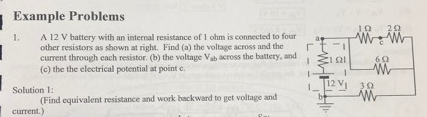# Example Problems1. A 12 V battery with an internal resistance of 1 ohm is connected to four aWW1229other resistors as shown at right. Find (a) the voltage across and theΓtcurrent through each resistor. (b) the voltage Vab across the battery. and(c) the the electrical potential at point c.6ΩSolution 1:(Find equivalent resistance and work backward to get voltage andcurrent.)

Question
3 views

Please Show all steps, thank you!help_outlineImage TranscriptioncloseExample Problems 1. A 12 V battery with an internal resistance of 1 ohm is connected to four aWW 1229 other resistors as shown at right. Find (a) the voltage across and the Γ t current through each resistor. (b) the voltage Vab across the battery. and (c) the the electrical potential at point c. 6Ω Solution 1: (Find equivalent resistance and work backward to get voltage and current.) fullscreen
check_circle

Step 1

To find the voltages and current in the given circuit, equivalent resistance is required.

First 1Ω and 2Ω are in series connection, their equivalent is 1+2= 3Ω. (fig:a)

This 3Ω and 6Ω are in parallel, their equivalent is (3*6)/(3+6)=2Ω. (fig:b)

Now, 1Ω, 2Ω and 3Ω are in series, their equivalent is, Req= 1+2+3 = 6Ω. (fig:c)

Step 2

Using Ohm’s Law, find the total current in the circuit, i.e.,

Step 3

Cuurent in each resistance is required to know to find the voltage across each resistance.

From the circuit, given,

As the internal resistance 1Ω and external resistance 3Ω ar...

### Want to see the full answer?

See Solution

#### Want to see this answer and more?

Solutions are written by subject experts who are available 24/7. Questions are typically answered within 1 hour.*

See Solution
*Response times may vary by subject and question.
Tagged in

### Physics